## Vertex Enumeration

A Convex Polyhedron is defined as the set of solutions to a system of linear inequalities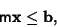where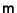is a Real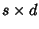Matrix and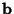is a Real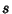-Vector. Givenand, vertex enumeration is the determination of the polyhedron's Vertices.

Avis, D. and Fukuda, K. A Pivoting Algorithm for Convex Hulls and Vertex Enumeration of Arrangements and Polyhedra.'' In Proceedings of the 7th ACM Symposium on Computational Geometry, North Conway, NH, 1991, pp. 98-104, 1991.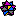Fukada, K. and Mizukosh, I. Vertex Enumeration Package for Convex Polytopes and Arrangements, Version 0.41 Beta.'' http://www.mathsource.com/cgi-bin/MathSource/Applications/Mathematics/0202-633.